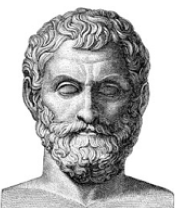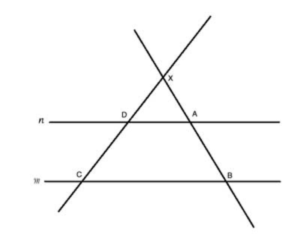## Thales of MiletusThales of Miletus was a Greek mathematician, astronomer and pre-Socratic philosopher from Miletus in Ionia, Asia Minor. He was one of the Seven Sages of Greece.

Thales used geometry to calculate the heights of pyramids and the distance of ships from the shore. He is the first person known to have used deductive reasoning applied to geometry, by deriving four corollaries to Thales’ theorem.Thales’s theorem

Thales’s theorem states that if A, B, and C are distinct points on a circle where the line AC is a diameter, then the angle ∠ABC is a right angle.Intercept theorem

The Intercept theorem provides the ratios between the line segments created when two parallel lines are intercepted by two intersecting lines.

It is sometimes called also “Thales’ Theorem”.

It states that if m and n are parallel lines, and XC and XB are two intersecting lines that intercept m and n, then the following relationship between the lengths of the line segments is true: